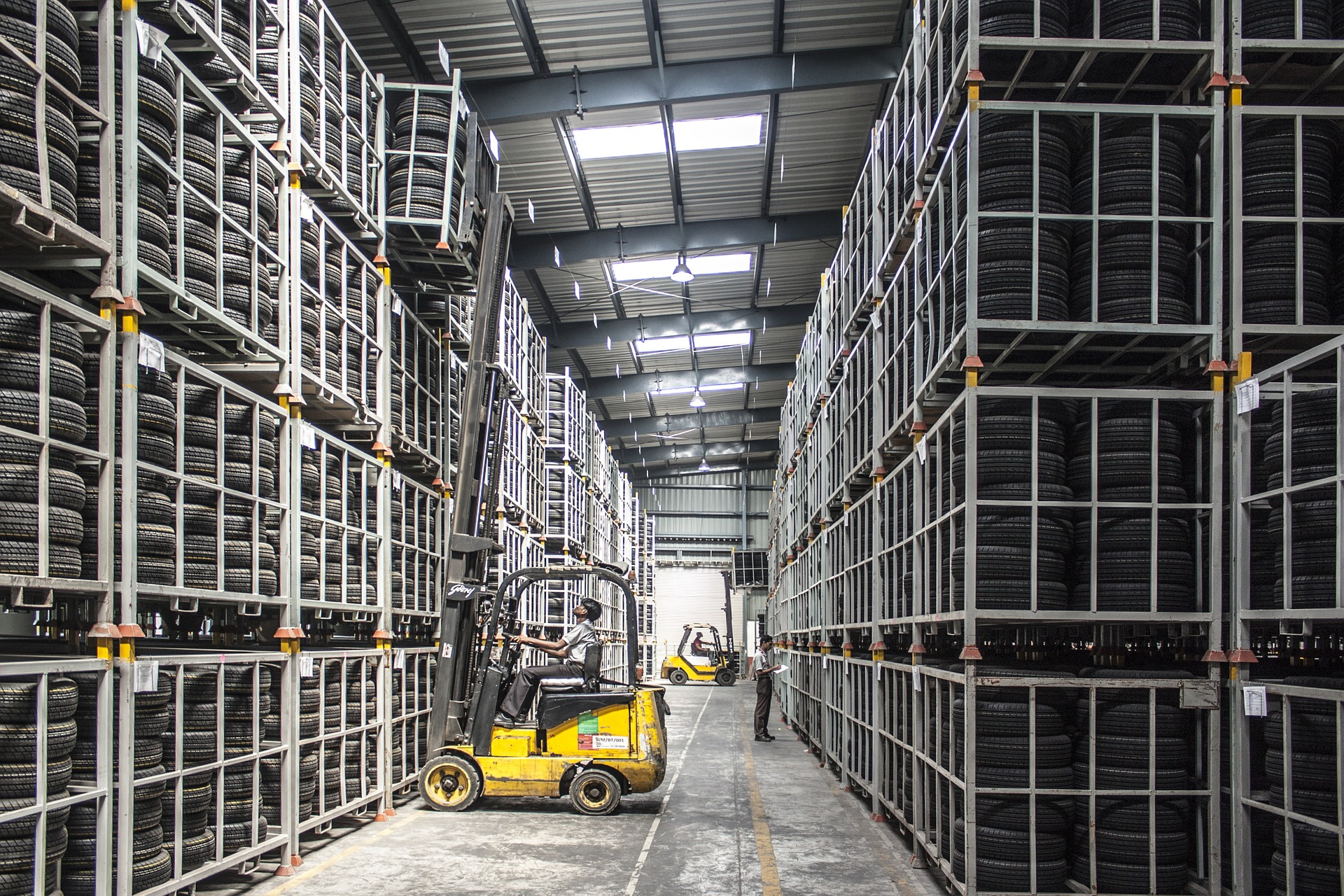# PyTorch - Python Deep Learning Neural Network API

Deep Learning Course 4 of 6 - Level: Intermediate

## Batch Norm in PyTorch - Add Normalization to Conv Net Layers

### video

expand_more chevron_left

### text

expand_more chevron_left

### Batch Normalization in PyTorch

Welcome to deeplizard. My name is Chris. In this episode, we're going to see how we can add batch normalization to a PyTorch CNN.Without further ado, let's get started.

### What is Batch Normalization?

In order to understand batch normalization, we need to first understand what data normalization is in general, and we learned about this concept in the episode on dataset normalization.

When we normalize a dataset, we are normalizing the input data that will be passed to the network, and when we add batch normalization to our network, we are normalizing the data again after it has passed through one or more layers.

One question that may come to mind is the following:

Why normalize again if the input is already normalized?

Well, as the data begins moving though layers, the values will begin to shift as the layer transformations are preformed. Normalizing the outputs from a layer ensures that the scale stays in a specific range as the data flows though the network from input to output.

The specific normalization technique that is typically used is called standardization. This is where we calculate a z-score using the mean and standard deviation.

$z=\frac{x-mean}{std}$

#### How Batch Norm Works

When using batch norm, the mean and standard deviation values are calculated with respect to the batch at the time normalization is applied. This is opposed to the entire dataset, like we saw with dataset normalization.

Additionally, there are two learnable parameters that allow the data the data to be scaled and shifted. We saw this in the paper: Batch Normalization: Accelerating Deep Network Training by Reducing Internal Covariate Shift

Note that the scaling given by $$\gamma$$ corresponds to the multiplication operation, and the sifting given by $$\beta$$ corresponds to the addition operation.

The Scale and sift operations sound fancy, but they simply mean multiply and add.

These learnable parameters give the distribution of values more freedom to move around, adjusting to the right fit.

The scale and sift values can be thought of as the slope and y-intercept values of a line, both which allow the line to be adjusted to fit various locations on the 2D plane.

### Adding Batch Norm to a CNN

Alright, let's create two networks, one with batch norm and one without. Then, we'll test these setups using the testing framework we've developed so far in the course. To do this, we'll make use of the nn.Sequential class.

Our first network will be called network1:

torch.manual_seed(50)
network1 = nn.Sequential(
nn.Conv2d(in_channels=1, out_channels=6, kernel_size=5)
, nn.ReLU()
, nn.MaxPool2d(kernel_size=2, stride=2)
, nn.Conv2d(in_channels=6, out_channels=12, kernel_size=5)
, nn.ReLU()
, nn.MaxPool2d(kernel_size=2, stride=2)
, nn.Flatten(start_dim=1)
, nn.Linear(in_features=12*4*4, out_features=120)
, nn.ReLU()
, nn.Linear(in_features=120, out_features=60)
, nn.ReLU()
, nn.Linear(in_features=60, out_features=10)
)


Our second network will be called network2:

torch.manual_seed(50)
network2 = nn.Sequential(
nn.Conv2d(in_channels=1, out_channels=6, kernel_size=5)
, nn.ReLU()
, nn.MaxPool2d(kernel_size=2, stride=2)
, nn.BatchNorm2d(6)
, nn.Conv2d(in_channels=6, out_channels=12, kernel_size=5)
, nn.ReLU()
, nn.MaxPool2d(kernel_size=2, stride=2)
, nn.Flatten(start_dim=1)
, nn.Linear(in_features=12*4*4, out_features=120)
, nn.ReLU()
, nn.BatchNorm1d(120)
, nn.Linear(in_features=120, out_features=60)
, nn.ReLU()
, nn.Linear(in_features=60, out_features=10)
)


Now, we'll create a networks dictionary that we'll use to store the two networks.

networks = {
'no_batch_norm': network1
,'batch_norm': network2
}


The names or keys of this dictionary will be used inside our run loop to access each network. To configure our runs, we can use the keys of the dictionary opposed to writing out each value explicity. This is pretty cool because it allows us to easily test different networks with one another simply by adding more networks to the dictionary. 😎

params = OrderedDict(
lr = [.01]
, batch_size = 
, num_workers = 
, device = ['cuda']
, trainset = ['normal']
, network = list(networks.keys())
)


Now, all we do inside our run loop is simply access the network using the run object that gives us access to the dictionary of networks. It's like this:

network = networks[run.network].to(device)


Boom! We're ready to test. The results look like this:

run epoch loss accuracy epoch duration run duration lr batch_size num_workers device trainset network
2 20 0.1636 0.9377 9.5200 196.9300 0.0100 1000 1 cuda normal batch_norm
2 19 0.1716 0.9335 9.5300 187.2900 0.0100 1000 1 cuda normal batch_norm
2 18 0.1757 0.9315 9.6400 177.6500 0.0100 1000 1 cuda normal batch_norm
2 17 0.1799 0.9311 9.5700 167.8900 0.0100 1000 1 cuda normal batch_norm
2 16 0.1865 0.9285 9.6200 158.2000 0.0100 1000 1 cuda normal batch_norm
2 15 0.1932 0.9266 9.6100 148.4700 0.0100 1000 1 cuda normal batch_norm
2 14 0.1978 0.9252 9.6800 138.7500 0.0100 1000 1 cuda normal batch_norm
2 13 0.2075 0.9214 9.5400 128.9700 0.0100 1000 1 cuda normal batch_norm
2 12 0.2087 0.9209 9.5500 119.3200 0.0100 1000 1 cuda normal batch_norm
2 11 0.2151 0.9197 9.5800 109.6600 0.0100 1000 1 cuda normal batch_norm
2 10 0.2240 0.9156 9.7100 99.9800 0.0100 1000 1 cuda normal batch_norm
1 20 0.2254 0.9150 9.6600 196.2000 0.0100 1000 1 cuda normal no_batch_norm
2 9 0.2304 0.9133 9.6600 90.1600 0.0100 1000 1 cuda normal batch_norm
1 19 0.2315 0.9130 9.6700 186.4500 0.0100 1000 1 cuda normal no_batch_norm

Batch norm smoked the competition and gave us the highest accuracy we've seen yet.

### quiz

expand_more chevron_leftDEEPLIZARD Message notifications

### resources

expand_more chevron_left

expand_more chevron_leftDEEPLIZARD Message notifications